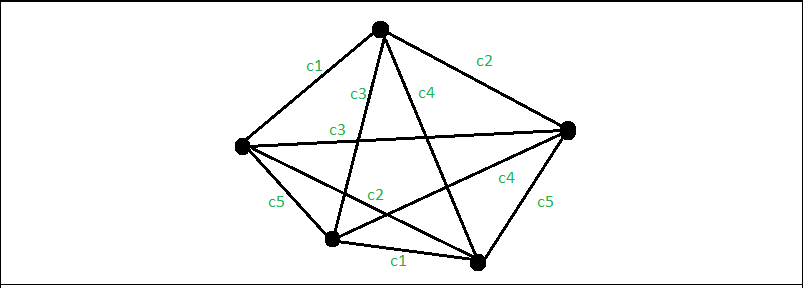# Vizing’s Theorem

In graph theory, Vizing’s theorem states that every simple undirected graph may be edge colored using a number of colors that is at most one larger than the maximum degree ‘d’ of the graph. In simple meaning this theorem states that the chromatic index of the simple graph can be either ‘d’ or ‘d’ +1. The maximum number of color needed for the edge coloring of the graph is called chromatic index.There are 5 vertices in the above graph G. Highest Degree is 4, but we need 5 colors, so that no edge shares the same color with any edge of the adjacent vertices, as you can see in the above graph. Therefore the required number of valid colors for this graph is equal to 5, which is ( ‘highest degree’ + 1 ).
Note: c1, c2, c3, c4 and c5 in the above diagram implies distinct colors.

Examples :

Input :
v = 3, e = 3
{{ 1, 2, -1 },
{ 2, 3, -1 },
{ 3, 1, -1 }};
Output :
3 colors needs to generate a valid edge coloring :

color between v(1): 1 and v(2): 2 is: color 1
color between v(1): 2 and v(2): 3 is: color 2
color between v(1): 3 and v(2): 1 is: color 3

## Recommended: Please try your approach on {IDE} first, before moving on to the solution.

Algorithm:

• After initializing the number of vertices and the number of edges, assign the vertex pair of every edge
• Calculate the maximum degree among all the vertices
• Color the graph edges according to the theorem
• Assign a color and then check its validity
• Check if any two adjacent edges have the same color, then increment the Color, goto flag and try next color
• Repeat till all the edges get it’s color according to the theorem
• Once done print the color of all the edges between the vertices

Below is the implementation of the above approach:

 `// C++ program to illustrate ` `// Vizing's Theorem ` `#include ` `using` `namespace` `std; ` ` `  `// Function to print the color of the edge ` `void` `colorEdge(``int` `edg[], ``int` `e) ` `{ ` `    ``int` `color; ` ` `  `    ``// Assign a color to every edge 'i'. ` `    ``for` `(``int` `i = 0; i < e; i++) { ` `        ``color = 1; ` `    ``flag: ` `        ``// Assign a color and ` `        ``// then check its validity. ` `        ``edg[i] = color; ` `        ``for` `(``int` `j = 0; j < e; j++) { ` `            ``if` `(j == i) ` `                ``continue``; ` ` `  `            ``// If the color of edges ` `            ``// is adjacent to edge i ` `            ``if` `((edg[i] == edg[j])||  ` `               ``(edg[i] == edg[j]) ||  ` `               ``(edg[i] == edg[j]) ||  ` `               ``(edg[i] == edg[j])) { ` ` `  `                ``// If the color matches ` `                ``if` `(edg[i] == edg[j]) { ` ` `  `                    ``// Increment the color, ` `                    ``// denotes the change in color. ` `                    ``color++; ` ` `  `                    ``// goes back, and assigns ` `                    ``// the next color. ` `                    ``goto` `flag; ` `                ``} ` `            ``} ` `        ``} ` `    ``} ` `} ` ` `  `// Function to assign Degree ` `void` `degree(``int` `v, ``int` `e, ``int` `edg[]) ` `{ ` `    ``int` `maximum = -1; ` `    ``int` `Deg[v + 1] = { 0 }; ` ` `  `    ``for` `(``int` `i = 0; i < e; i++) { ` `        ``Deg[edg[i]]++; ` `        ``Deg[edg[i]]++; ` `    ``} ` ` `  `    ``// To find the maximum degree. ` `    ``for` `(``int` `i = 1; i <= v; i++) { ` `        ``if` `(maximum < Deg[i]) ` `            ``maximum = Deg[i]; ` `    ``} ` ` `  `    ``// color the edges of the graph. ` `    ``colorEdge(edg, e); ` `    ``cout << maximum + 1 << ``" colors needs to generate"` `         ``<< ``" a valid edge coloring :\n"``; ` ` `  `    ``for` `(``int` `i = 0; i < e; i++) ` `        ``cout << ``"\ncolor between v(1): "` `<< edg[i] << ``" and v(2): "` `             ``<< edg[i] << ``" is: color "` `<< edg[i]; ` `} ` ` `  `// Driver Code ` `int` `main() ` `{ ` ` `  `    ``// initialize the number ` `    ``// of vertexes of the graph ` `    ``int` `v = 4; ` `    ``// initialize the number ` `    ``// of edges of the graph ` `    ``int` `e = 5; ` ` `  `    ``// initialize the vertex ` `    ``// pair of every edge in a graph ` `    ``int` `edg[e] = { { 1, 2, -1 }, ` `                      ``{ 2, 3, -1 }, ` `                      ``{ 3, 4, -1 }, ` `                      ``{ 4, 1, -1 }, ` `                      ``{ 1, 3, -1 } }; ` ` `  `    ``degree(v, e, edg); ` `    ``return` `0; ` `} `

Output:

```4 colors needs to generate a valid edge coloring :

color between v(1): 1 and v(2): 2 is: color 1
color between v(1): 2 and v(2): 3 is: color 2
color between v(1): 3 and v(2): 4 is: color 1
color between v(1): 4 and v(2): 1 is: color 2
color between v(1): 1 and v(2): 3 is: color 3
```

GeeksforGeeks has prepared a complete interview preparation course with premium videos, theory, practice problems, TA support and many more features. Please refer Placement 100 for details

My Personal Notes arrow_drop_upCheck out this Author's contributed articles.

If you like GeeksforGeeks and would like to contribute, you can also write an article using contribute.geeksforgeeks.org or mail your article to contribute@geeksforgeeks.org. See your article appearing on the GeeksforGeeks main page and help other Geeks.

Please Improve this article if you find anything incorrect by clicking on the "Improve Article" button below.

Article Tags :
Practice Tags :

1

Please write to us at contribute@geeksforgeeks.org to report any issue with the above content.